# The Jubilee Edit

Enjoy 15% off 10 balms, 25% off 30 balms and 40% off 40 balms or 50% off 50 balms in our Jubilee Balm Bundles.
Or spend £30 and buy any other item below at 1952 prices (around £1 in today's money!) with the code JUBILEE. Choose from our sumptuous I Want To Feel sets, our delicious Relaxation sets, any of our exquisite home candles, our Sleep Essentials set and our De Stress Wash and Lotion Duo set.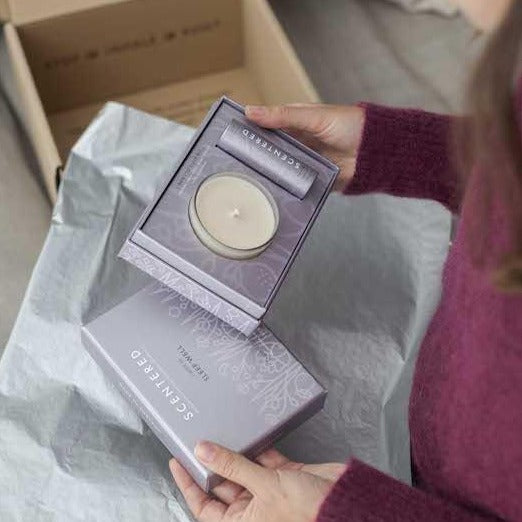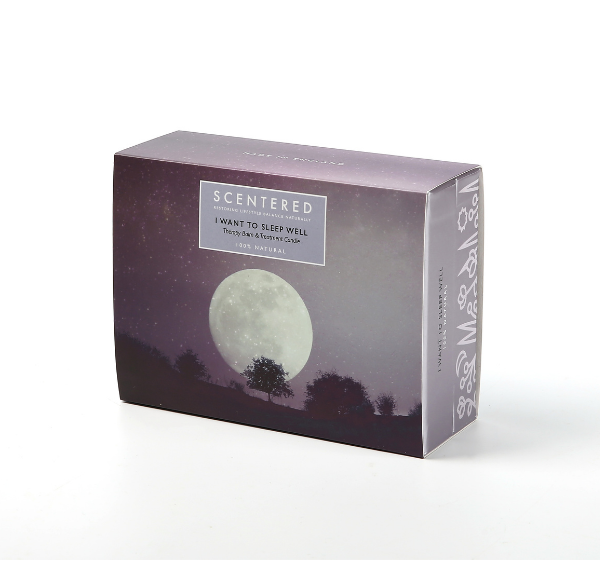### I\$ W\$a\$n\$t\$ T\$o\$ S\$L\$E\$E\$P\$ W

£29.50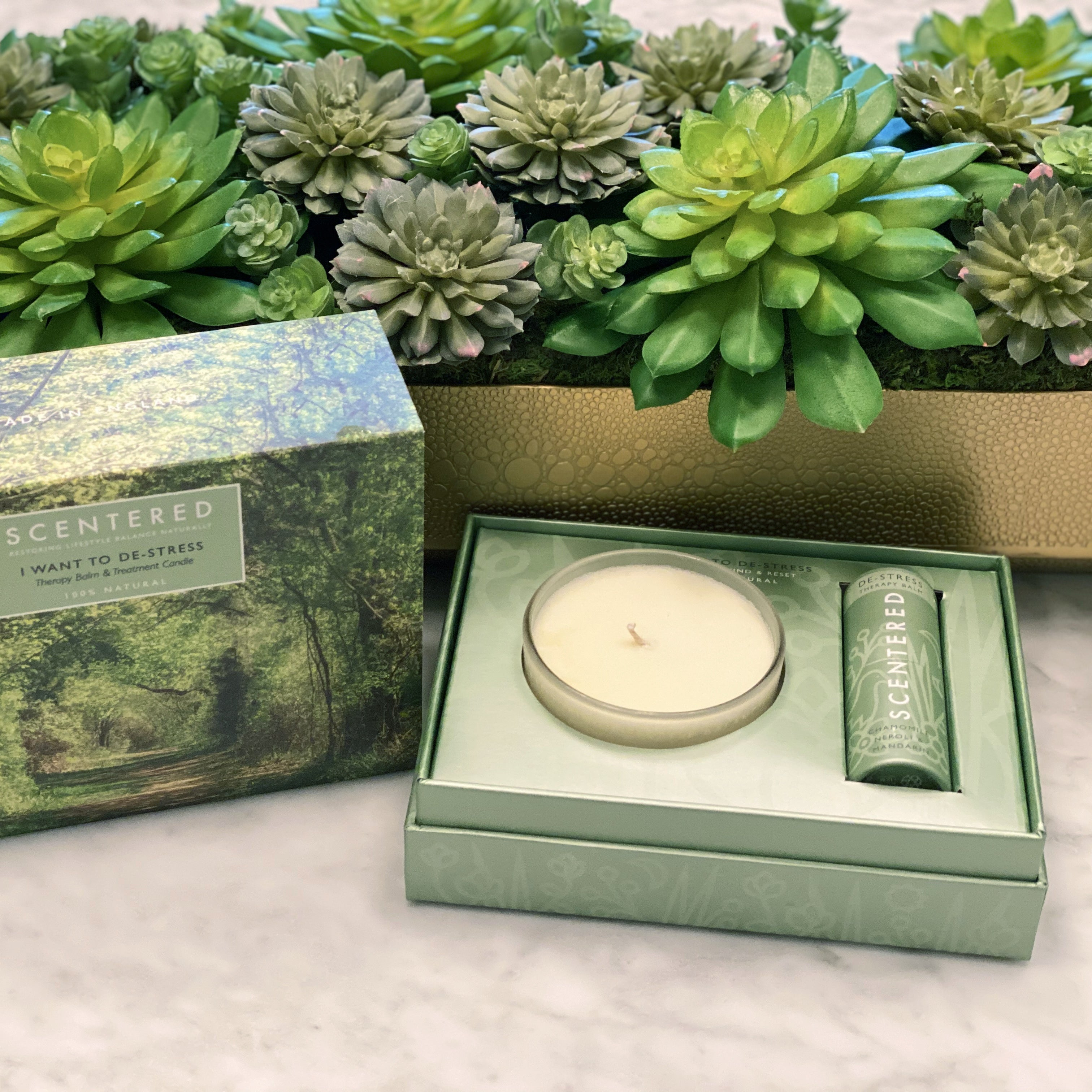### I\$ W\$a\$n\$t\$ T\$o\$ D\$E\$-S\$T\$R\$

£29.50### D\$E\$-S\$T\$R\$E\$S\$S\$ H\$o\$m\$e\$ A\$r\$o\$m

De £16.95### E\$S\$C\$A\$P\$E\$ H\$o\$m\$e\$ A\$r\$o\$m\$a

De £16.95### S\$L\$E\$E\$P\$ W\$E\$L\$L\$ H\$o\$m\$e\$ A\$r\$o\$

De £16.95### L\$O\$V\$E\$ H\$o\$m\$e\$ A\$r\$o\$m\$a\$t

De £16.95### I\$ W\$a\$n\$t\$ T\$o\$ E\$S\$C\$A\$

£29.50### H\$o\$m\$e\$ S\$p\$a\$ D\$e\$ S\$t\$r\$e\$s\$s\$

£29.50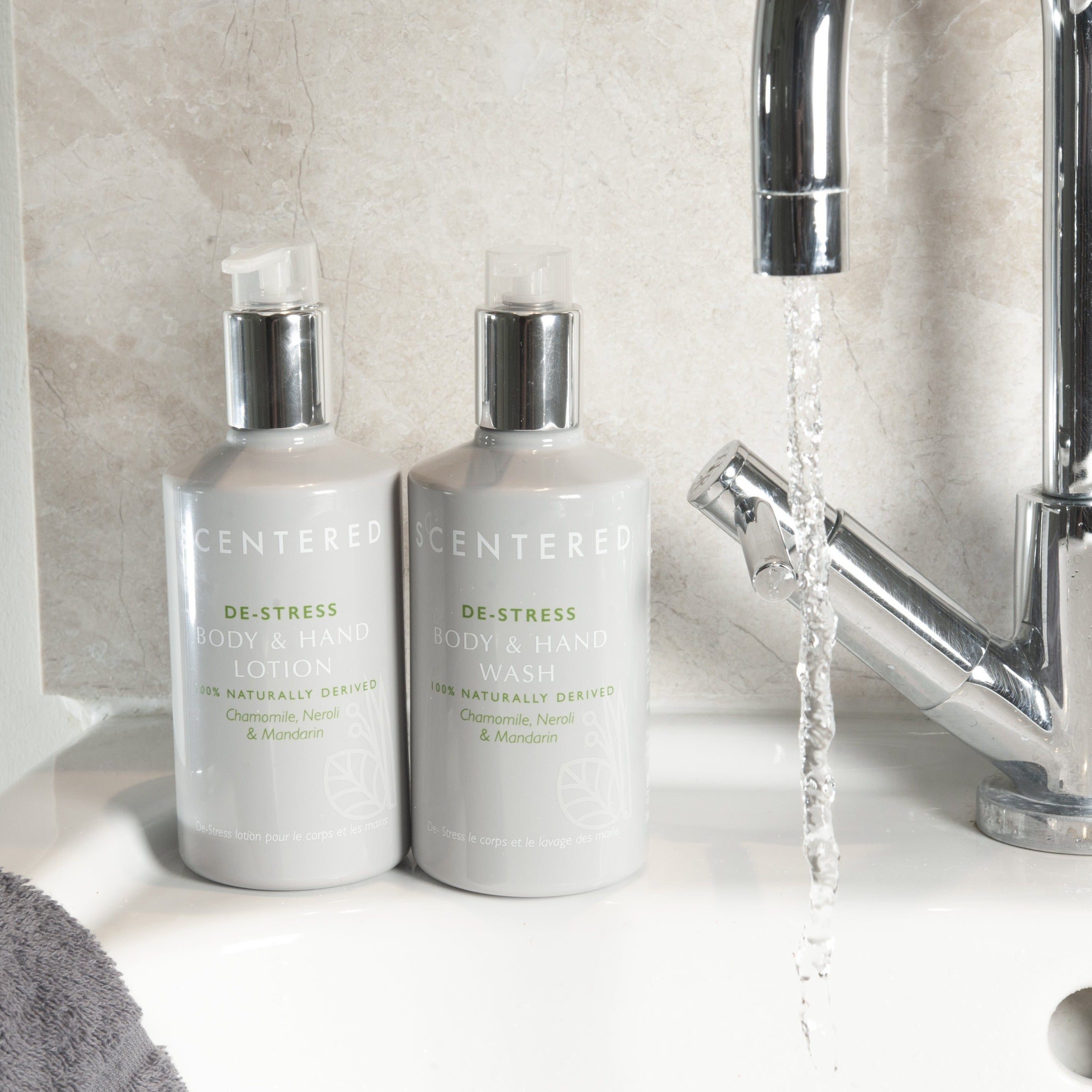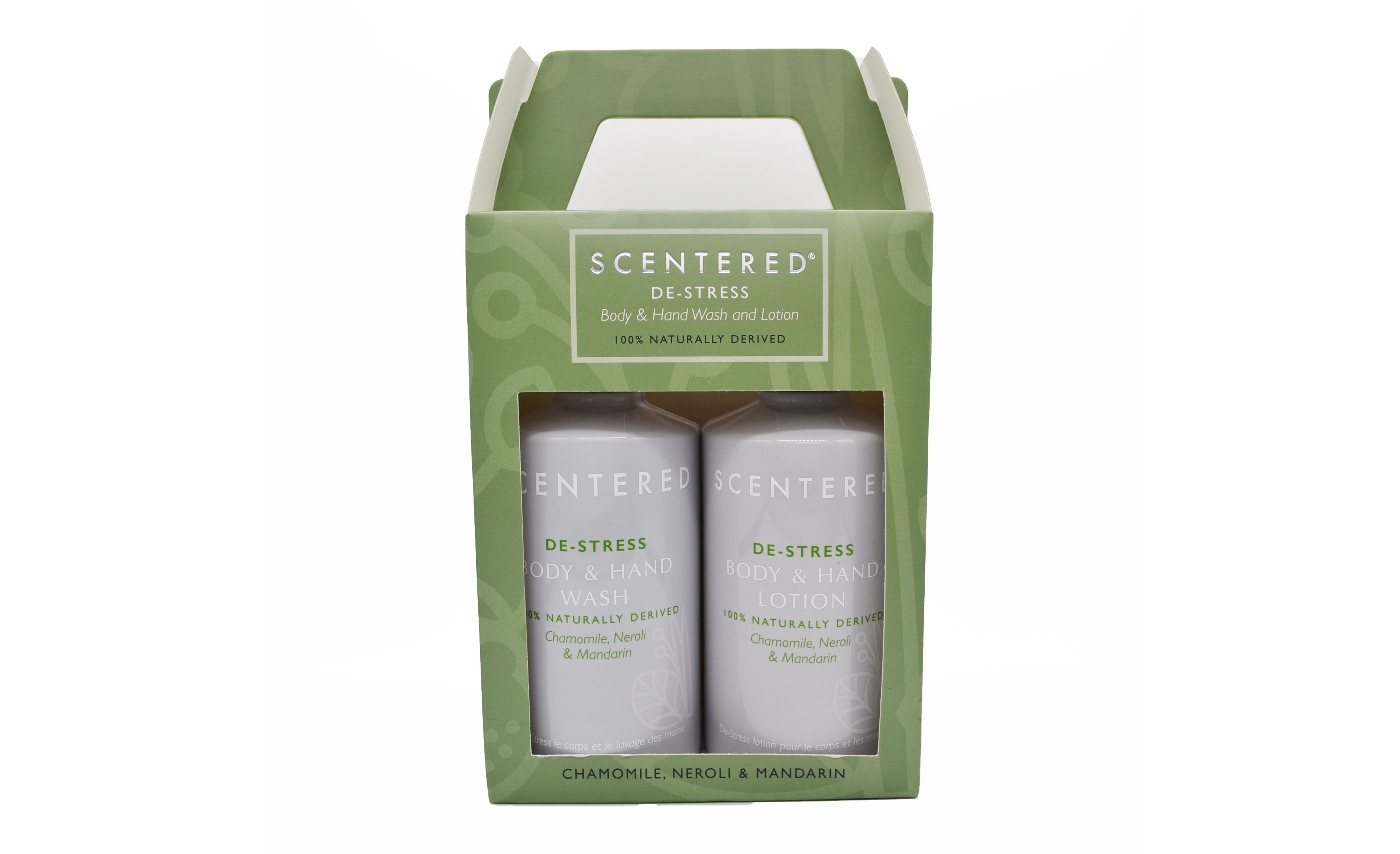### D\$E\$-S\$T\$R\$E\$S\$S\$ W\$a\$s\$h\$

£29.00### L\$u\$x\$u\$r\$y\$ W\$e\$l\$l\$b\$e\$i\$n\$g\$ S\$l\$

£29.00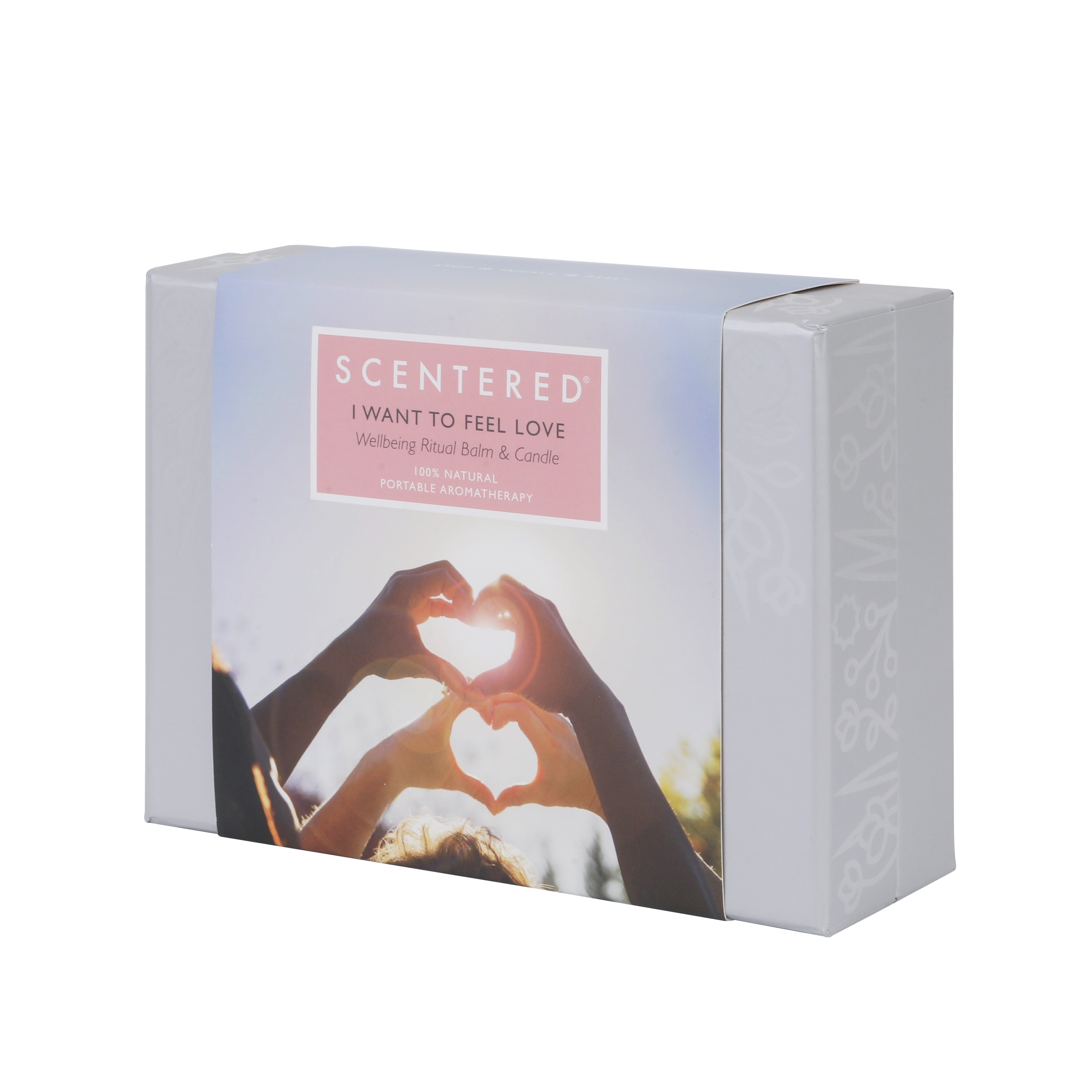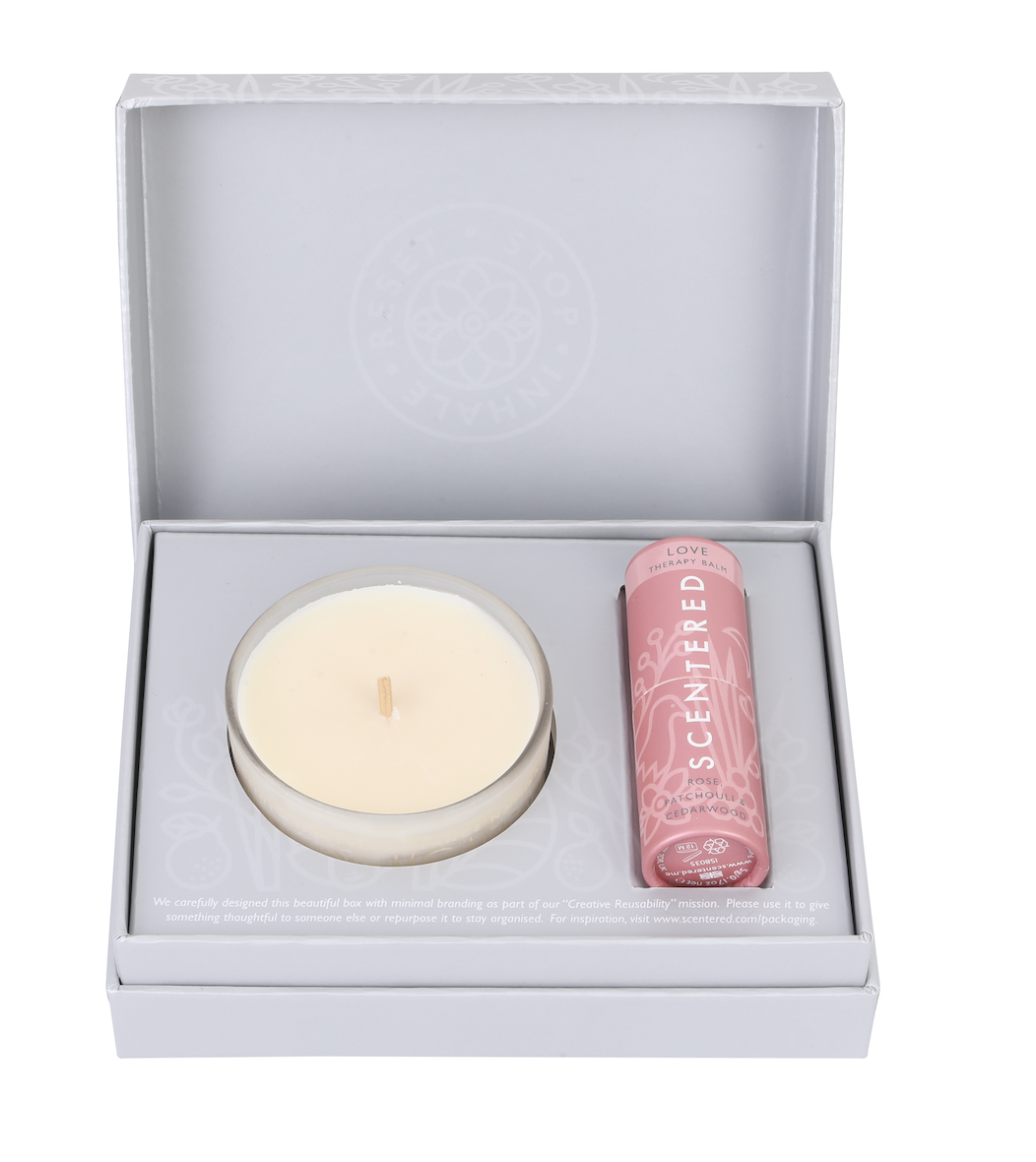### I\$ W\$a\$n\$t\$ T\$o\$ F\$e\$e\$l\$ L\$

£29.50£29.50